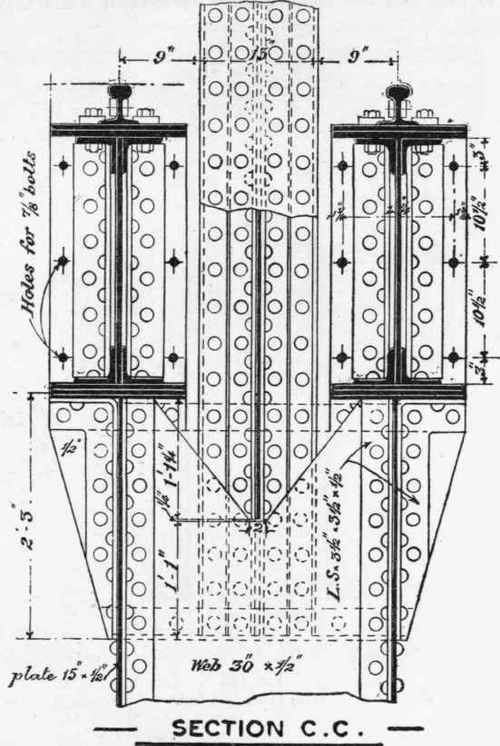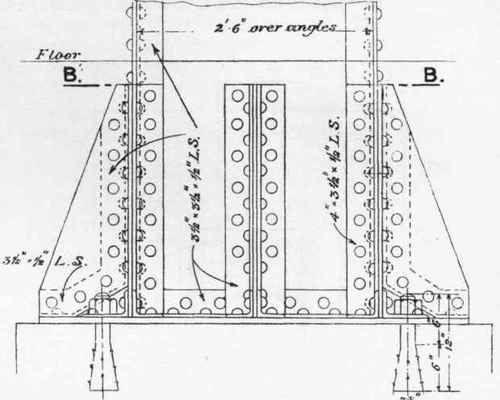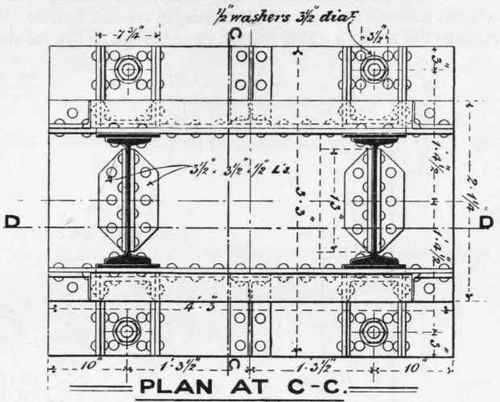The method of effecting the connection between the upper and lower sections of the column at the level of the traveller girder is shown in Figs. 197 and 198, which is a variation from the design shown in Figs. 189 and 190. The normal section of the lower portion of the column is shown in Fig. 200, together with the details of the stiffener shown in plan in Fig. 200, and in elevation in Fig. 201. Details of the base of this column are shown in Figs. 202, 203, 204, and 205, with lewis bolts securing the column to a masonry bedstone.Fig. 189. Scale ¾ inch = 1 foot.Fig. 190. Scale ¾ inch = 1 foot.Fig. 191. Scale \ inch = 1 foot.Fig. 192. Scale ¾ inch = 1 foot.Fig. 193. Scale \ inch = 1 foot.Fig. 194. Scale \ inch = 1 foot.Fig. 195. Scale ¾ inch = 1 foot.Fig. 196. Scale ¾ inch = 1 foot.

A column, of which the simple elementary type is shown in Fig. 157, is shown in detail in Figs. 206 to 215 inclusive.

This column consists, in its lower portion, of two rolled joists strengthened with flange plates, and connected together into one member by the parallel system of flat bar lattices, shown in elevation in Figs. 206 and 207, which are side elevations of the column at the base and cap respectively. The front elevation is given in Figs. 208 and 209, also at the base and cap respectively. The normal section of the lower portion is given in Fig. 210. It will be seen from the figure that the flat bar braces are slightly bent to pass over one another at the intersection, where they are riveted together, while the flat bar bracing, consisting of 4" X ⅝" flats riveted together at the meeting face, is intended to prevent any tendency to a corkscrew twist in the rolled joist members of the column. The weight-bearing section is also seen to consist of two rolled joists 16 inches deep at 62 lbs., each with two 8" X ½" plates riveted on.Fig. 197. Scale ¾ inch = 1 foot.Fig. 198. Scale ¾ inch = 1 foot.

A sectional elevation of the base is shown in Fig. 211, on the line DD of Fig. 212.Fig. 199. Scale ¾ inch = 1 foot.Fig. 200. Scale ¾ inch = 1 foot.Fig. 201. Scale ¾ inch = 1 foot.

A sectional plan of the column on the line CC of Fig. 206, looking down, is given in Fig. 212, from which and Fig. 206 it will be seen that the column is held down by four holding-down bolts, which in this case pass through a bed of concrete.Fig. 202. Scale ¾ inch = 1 foot.Fig. 203. Scale ¾ inch = 1 foot.Fig. 204. Scale ¾ inch - 1 foot.Fig. 205 Scale ¾ inch = 1 foot.

The column carries two lines of traveller girders, while the upper section, continued above the traveller girder seatings, carries a roof load. The connection between the upper and lower sections of the column, at the level of the traveller girders, is shown in Figs. 207, 209, 213, and 214. Fig. 213 is a sectional plan, looking down, on the line AA of Fig. 207, and shows the upper faces of the traveller girder seatings.Fig. 206. Scale ¾ inch = 1 foot.Pig. 207. Scale ¾ inch = 1 foot.

Fig. 214 is a vertical section on the line FF of Fig. 213. It will be seen that the normal section of the upper portion of the column is of the type shown in Fig. 156, and a plan of its cap is shown in Fig. 215.Fig. 208. Scale ¾ inch = 1 foot.

It will be observed that the load arising from the upper section is distributed equally over the two members of the lower section by means of the plates and angles forming the cap and seatings of the traveller girders. This member has to act, therefore, as a girder, being subject to transverse and shearing stresses, while the loads arising from the traveller girders are transmitted directly down the two principal members of the lower column. A column of somewhat complex type will now be described, the arrangement of the details and connections being determined by the conditions of the site and the peculiarities of loading.Fig. 209. Scale ¾ inch = 1 foot.Fig. 210. Scale \ inch = 1 foot.Fig. 211. Scale ¾ inch = 1 foot.Fig. 212 (Scale ¾ inch = 1 foot).Fig. 213 (Scale ¾ inch = 1 foot).Fig. 214.

Scale ¾ inch = 1 foot.Fig. 215. Scale ¾ inch = 1 foot.